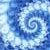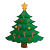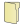Games
Problems
Go Pro!

# Number Theory

Pro Problems > Math > Number and Quantity > Number Theory

# Featured Pro ProblemsWithout a calculator find a nine digit number that meets the criteria of the problemA math problem involving Shrek wanting Fiona's telephone numberStrings of red lights and green lights on my Christmas tree - a Christmas word problemA diophantine equation - quadratic in two variablesUse divisibility rules to determine the number with missing digitsGiven two pieces of information about the three digit number, determine all possible values.Solve an algebra problem involving a defined binary operation (the hashtag or pound symbol)Find the sum of two binary numbers, write the answer in base ten# Full Directory Listing

Crossing Out Numbers, How many zeroes?, Nine Digit Number, Pyramid of BlocksLike us on Facebook to get updates about new resources